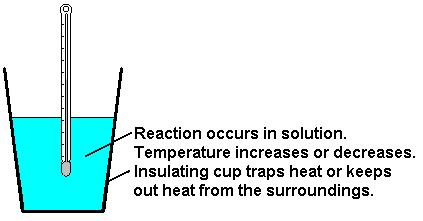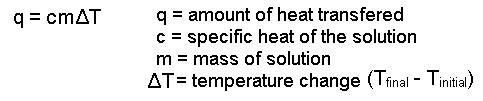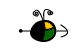Calorimetry

If we run an exothermic reaction in solution in a calorimeter, the heat produced by the reaction is trapped in the calorimeter and increases the temperature of the solution. If we run an endothermic reaction, the heat required by the reaction is removed from the solution and the temperature of the solution decreases.We can calculate the amount of heat absorbed by the solution or the amount of heat removed from the solution with the following equation:Note:

• When heat is absorbed by the solution, q for the solution has a positive value. This means that the reaction produces heat for the solution to absorb and q for the reaction is negative.

• When heat is absorbed from the solution q for the solution has a negative value. This means that the reaction absorbs heat fron the solution, the reaction is endothermic, and q for the reaction is positive.

Top